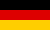Bri's worldelectronics, programming and more

deutschNumeric Laplace Transform inversion for control theory applications

In control engineering the Laplace transform of the transfer function is used to describe the transmission behaviour of control circuits and control loops. The advantage of this method is that in the time domain difficult to calculate mathematical expressions are easy to calculate in the Laplace domain, e.g. a convolution in the time domain is a multiplication in the Laplace domain.

The Laplace transform inversion is ised to make a function in the time domain from a transfer function in the Laplace domain. A computer algebra system like Matlab or Maxima can be used to calculate a analytical solution. Without access to a computer algebra system a numeric Laplace transform inversion is a good alternative.

There are a lot of methods for the numerical inverse Laplace transform. These methods are based on FFT series expansion, Laguerre polynomials or other algorithms. For applications in the control theory FFT based methods are particularly favourable because these methods return a good result for periodic processes.

Algorithms for numeric Laplace transform inversion

The here used source code of the algorithms for the numerical inverse Laplace transformation can be watched here:

Algorithms for numeric Laplace transform inversion

Numeric Laplace transform inversion algorithm comparison

Function Transfer function K T D

Step response analytical

Method 1 Method 2 End time DEQ time step